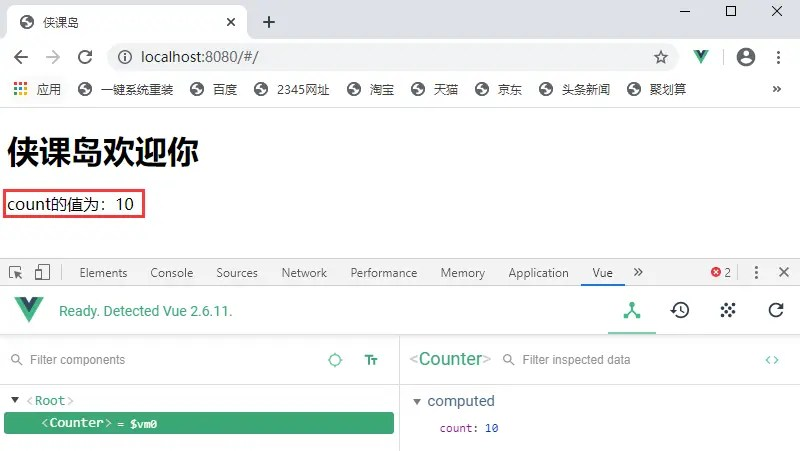# State in Vuex

Posted May 27, 20203 min read

state is the basic data in `Vuex`, and what is stored on state is the so-called state. When the state is not used, initialize it directly in `data`, and after we have the state, we will transfer the data on the data to the state.

#### Single state tree

`Vuex` uses a single state tree, that is, an object contains all state data. In other words, if we define an instance of a store, then there is only one state in this store instance. state as a constructor option defines all the basic state parameters we need.

The single state tree allows us to directly locate any specific state segment, and can easily take a snapshot of the entire current application state during debugging.

#### Get Vuex status in Vue component

The easiest way to read the state from the store instance is to return a certain state in the calculated properties.

##### Example:

For example, we define a count attribute in the state and assign it a value of 10. The contents of the `store.js` file are as follows:

``````import Vue from 'vue' //import vue
import Vuex from 'vuex' //import vuex

Vue.use(Vuex)

const state = {
count:10
}

export default new Vuex.Store({
state
})``````

Then create a Counter component and return the count in the component, as shown below:

``````const Counter = {
template:`<div> count is:{{count}} </div>`,
computed:{
count() {
return store.state.count //Return the count state of the store instance
}
}
}``````

Whenever `store.state.count` changes, it will re-calculate the count attribute and refresh the interface.

This mode depends on the global administrator store. If there are more modules, every module or page needs to import the store as long as it uses the data in this state. This operation is really a bit uncomfortable. So the following solution appeared.

Vuex provides a mechanism to "inject" state from the root component into each subcomponent through the store option:

``````new Vue({
el:'#app',
store, //The root component injects the store instance into all local child components through the store option
//Subassembly
components:{Counter},
template:`
<div class = "app">
<counter> </counter>
</div>
`
})``````

The content of the index.html file of the Vue project is as follows:

``````<! DOCTYPE html>
<html>
<meta charset = "utf-8">
<title> Xia Kedao </title>
<body>
<h1> Welcome to Xia Kedao </h1>
<div id = "app"> </div>
</body>
</html>``````

Finally, we use `npm run dev` to run the project. The output in the browser is shown in the figure below. If we change the value of count in the state, the page will automatically refresh:#### mapState helper function

When a component needs to acquire multiple states, declaring these states as calculated attributes will be somewhat repetitive and redundant. In order to solve this problem, we can use the `mapState` helper function to help us generate calculated attributes, allowing you to press a few keys.

##### Example:

Before using the `mapState` function, you need to introduce it first:

``import {mapState} from 'vuex'``

It can be used only after being introduced. It has two uses, and can accept an object or an array.

Object usage is as follows:

``````import {mapState} from 'vuex'

export default {
//Both of the following can be written
computed:mapState({
//Each property function in the component will get a default parameter state, and then directly get its properties through state
count:state => state.count,
//'count' maps directly to the count in the state object, equivalent to this. \$store.state.count
count:'count'
})
}``````

The array usage is as follows:

``````//When the name of the calculated attribute of the map is the same as the name of the child node of state, we can also pass a string array to mapState.
export default {
computed:mapState([//array
'count'
])
}``````